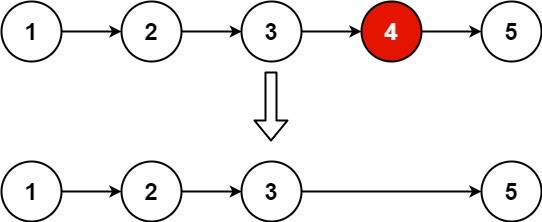# 2022-05-19

## ​876. Middle of the Linked List​

### Description

Given the `head` of a singly linked list, return the middle node of the linked list.
If there are two middle nodes, return the second middle node.
Example 1:Output: [3,4,5]
Explanation: The middle node of the list is node 3.
Example 2:Output: [4,5,6]
Explanation: Since the list has two middle nodes with values 3 and 4, we return the second one.
Constraints:
• The number of nodes in the list is in the range `[1, 100]`.
• `1 <= Node.val <= 100`

### Solution

#### Approach #0: Single Pointer

/**
* type ListNode struct {
* Val int
* Next *ListNode
* }
*/
count := 0
for first.Next != nil {
count++
first = first.Next
}
for i := 0; i < count/2+count%2; i++ {
second = second.Next
}
return second
}

#### Approach #1: Fast and Slow Pointer

/**
* type ListNode struct {
* Val int
* Next *ListNode
* }
*/
for second != nil && second.Next != nil {
first = first.Next
second = second.Next.Next
}
return first
}

#### Approach #2: Output to Array

/**
* type ListNode struct {
* Val int
* Next *ListNode
* }
*/
var l []*ListNode
}
return l[len(l)/2]
}

## ​19. Remove Nth Node From End of List​

### Description

Given the `head` of a linked list, remove the `nth` node from the end of the list and return its head.
Example 1:Input: head = [1,2,3,4,5], n = 2
Output: [1,2,3,5]
Example 2:
Input: head = , n = 1
Output: []
Example 3:
Input: head = [1,2], n = 1
Output: 
Constraints:
• The number of nodes in the list is `sz`.
• `1 <= sz <= 30`
• `0 <= Node.val <= 100`
• `1 <= n <= sz`
Follow up: Could you do this in one pass?

### Solution

#### Approach #0

/**
* type ListNode struct {
* Val int
* Next *ListNode
* }
*/
func removeNthFromEnd(head *ListNode, n int) *ListNode {
count := 0
for first != nil {
count++
first = first.Next
}
for i := 0; i < count-n; i++ {
second = second.Next
}
second.Next = second.Next.Next
}

#### Approach #1

/**
* type ListNode struct {
* Val int
* Next *ListNode
* }
*/
func removeNthFromEnd(head *ListNode, n int) *ListNode {
var nodeList []*ListNode
for cur := newHead; cur != nil; cur = cur.Next {
nodeList = append(nodeList, cur)
}
prev := nodeList[len(nodeList)-n-1]
prev.Next = prev.Next.Next
}

#### Approach #2

/**
* type ListNode struct {
* Val int
* Next *ListNode
* }
*/
func removeNthFromEnd(head *ListNode, n int) *ListNode {